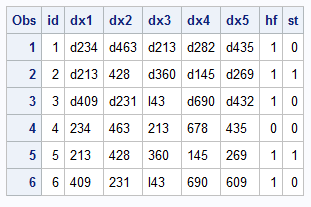## ifn susbtrn for multiple lengths

I have the following code:

data a (drop=i);

set b;

array x{*} dx:;

hf=0;

do i=1 to dim(x);

hf=ifn(substrn(x{i}, 1, 3) in('428', 'I43'),1,hf);

end;

run;

data a (drop=i);

set b;

array x{*} dx:;

hf=0;

do i=1 to dim(x);

hf=ifn(substrn(x{i}, 1, 4) in('d282', 'd432'),1,hf);

end;

run;

Is there is a way to combine the two codes into one code given that I am requiring different lengths in the substrn statement, (For example 3 in the first and 4 in the second).

I am looking to get a code that would be something similar to:

hf=ifn(substrn(x{i}, 1, 3) in('428', 'I43'),1,hf) or hf=ifn(substrn(x{i}, 1, 4) in('d282', 'd432'),1,hf);

Is it possible to have such statement?

Thanks

1 ACCEPTED SOLUTION

Accepted Solutionsart297
Opal | Level 21

## Re: ifn susbtrn for multiple lengths

Not sure what you are trying to do but, if i do understand correctly, the following should work:

``````data b;
input (dx1-dx5) (\$);
datalines;
d234 d463 d213 d282 d435
d213 428 d360 d145 d269
d409 d231 I43 d690 d432
234 463 213 678 435
213 428 360 145 269
409 231 I43 690 609
;
data a (drop=i);
set b;
array x{*} dx:;
hf=0;
do i=1 to dim(x);
hf=ifn(substrn(x{i}, 1, 3) in('428', 'I43')
or
substrn(x{i}, 1, 4) in('d282', 'd432'),
1,hf);
end;
run;
``````

Art, CEO, AnalystFinder.com

7 REPLIES 7

## Re: ifn susbtrn for multiple lengths

have you tried:

if hf=ifn(substrn(x{i}, 1, 3) in('428', 'I43'),1,hf);

else if hf=ifn(substrn(x{i}, 1, 4) in('d282', 'd432'),1,hf);

## Re: ifn susbtrn for multiple lengths

Thank you VDD, the statement is not correct and would generate an error (no matching if-then clause)

## Re: ifn susbtrn for multiple lengths

Thank you for sharing the link. If I understand correctly, they did not use the else statement with "ifn" so I am not sure it is applicable here. Maybe one of the advisors can help (@RW9)art297
Opal | Level 21

## Re: ifn susbtrn for multiple lengths

Not sure what you are trying to do but, if i do understand correctly, the following should work:

``````data b;
input (dx1-dx5) (\$);
datalines;
d234 d463 d213 d282 d435
d213 428 d360 d145 d269
d409 d231 I43 d690 d432
234 463 213 678 435
213 428 360 145 269
409 231 I43 690 609
;
data a (drop=i);
set b;
array x{*} dx:;
hf=0;
do i=1 to dim(x);
hf=ifn(substrn(x{i}, 1, 3) in('428', 'I43')
or
substrn(x{i}, 1, 4) in('d282', 'd432'),
1,hf);
end;
run;
``````

Art, CEO, AnalystFinder.comdata_null__

## Re: ifn susbtrn for multiple lengths

Seems like you're making this too hard.  It would be helpful to see your input data.  I borrowed from @art297 please indicate if that assumption is correct.

``````data b;
id + 1;
array dx \$8;
input dx[*];
array _hf \$8 _temporary_ ('428' 'I43' 'd282' 'd432');
array _st \$8 _temporary_ ('d269' '428');
hf=0; st=0;
do i = 1 to dim(dx);;
hf + dx[i] in _hf);
st + dx[i] in _st);
end;
hf = hf gt 0;
st = st gt 0;
drop i;
label hf='Heart Failure' st='Stroke';
datalines;
d234 d463 d213 d282 d435
d213 428 d360 d145 d269
d409 d231 I43 d690 d432
234 463 213 678 435
213 428 360 145 269
409 231 I43 690 609
;;;;
run;
proc print;
run;``````## Re: ifn susbtrn for multiple lengths

Thank you!

Discussion stats
• 7 replies
• 748 views
• 4 likes
• 4 in conversation